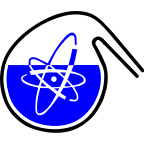#RadChem 2018

13-18 May 2018
Casino Conference Centre
Europe/Prague timezone

## Vertical Migration of Radionuclides in Soil Samples from Pripyat

17 May 2018, 09:30
15m
Red Hall (Casino Conference Centre)

### Red Hall

#### Casino Conference Centre

Reitenbergerova 4/95, Mariánské Lázně, Czech Republic
Verbal Radionuclides in the Environment, Radioecology

Mr Daniel Heine

### Description

Daniel Heine$^1$, Clemens Walther$^1$, Stefan Bister$^1$
$^1$Institute of Radioecology and Radiation Protection, Leibniz Universität Hannover,
30167, Germany
Keywords: Pripyat, Chernobyl, contamination, migration in soil
Presenting author e-mail: heine@irs.uni-hannover.de

The accident of Chernobyl led to significant contamination in large regions of Europe, particularly in Ukraine, Belarus and Russia. In the Chernobyl exclusion zone (CEZ), which includes a 2200 km$^2$ large area around the Chernobyl nuclear power plant, the highest amount of radioactivity was distributed. The decrease of surface activity concentrations is governed not only by physical decay but also by vertical migration. In this study the vertical distribution of $^{137}$Cs, $^{90}$Sr, $^{241}$Am and plutonium in upper soil layers from the exclusion zone was investigated to get information on the retention mechanisms of these nuclides. Therefore, several drill cores of 30 cm length were collected in the city of Pripyat, which is about 4 km away from the nuclear power plant. Each drill core was cut into 10 layers of different thicknesses. Due to their half-lifes $^{137}$Cs (T$_{1/2}$=30,17 a) and $^{90}$Sr (T$_{1/2}$=28,78 a) still dominate the contamination in this region. Because of their relevance to long-term considerations the different isotopes of plutonium ($^{238}$Pu, $^{239}$Pu, $^{240}$Pu) as well as $^{241}$Am being the daughter of $^{241}$Pu were also investigated.

Methods and Measurements

In the first step, each sample was analyzed by γ-spectrometry to determine the amount of $^{137}$Cs and $^{241}$Am by their characteristic γ-lines ($^{137}$Cs: E$_γ$=661,7 keV; $^{241}$Am: E$_γ$=59,54 keV). In the next steps, the soil samples have been ashed and plutonium and strontium were chemically separated. For the detection of plutonium α-spectrometry was used. The $^{90}$Sr measurements were performed by liquid scintillation counting (LSC).

Results

Figure 1 shows the specific activity of $^{241}$Am, $^{137}$Cs, $^{90}$Sr and the three plutonium isotopes in different depths of one drill core. While the absolute specific activities in layers of equal depth differ considerably, the decrease follows similar slopes in all cores and all nuclide below the 15 cm. Each nuclide is detected down to 30 cm depth.

Figure 1: Specific activities in soil layers.

Important information on the different migration behavior is obtained from the specific activity ratios of different nuclides (Figure 2).

Figure 2: Ratio of $^{241}$Am and $^{239+240}$Pu activities for two cores.

From the decrease in the ratio of $^{241}$Am and $^{239+240}$Pu activities migration rates of plutonium and americium are deduced by a simple model.

References
[ 1 ] MANDEL, M.; POTTGIESSER, S.; Plutonium distribution in soil from northern Ukraine. Leibniz Universität Hannover, Institute for Radioecology and Radiation Protection; 2016
[ 2 ] BROZYNSKI, P.; Migration of radionuclides in soil samples from northern Ukraine. Leibniz Universität Hannover, Radioecology and Radiation Protection; 2016

### Presentation Materials

There are no materials yet.